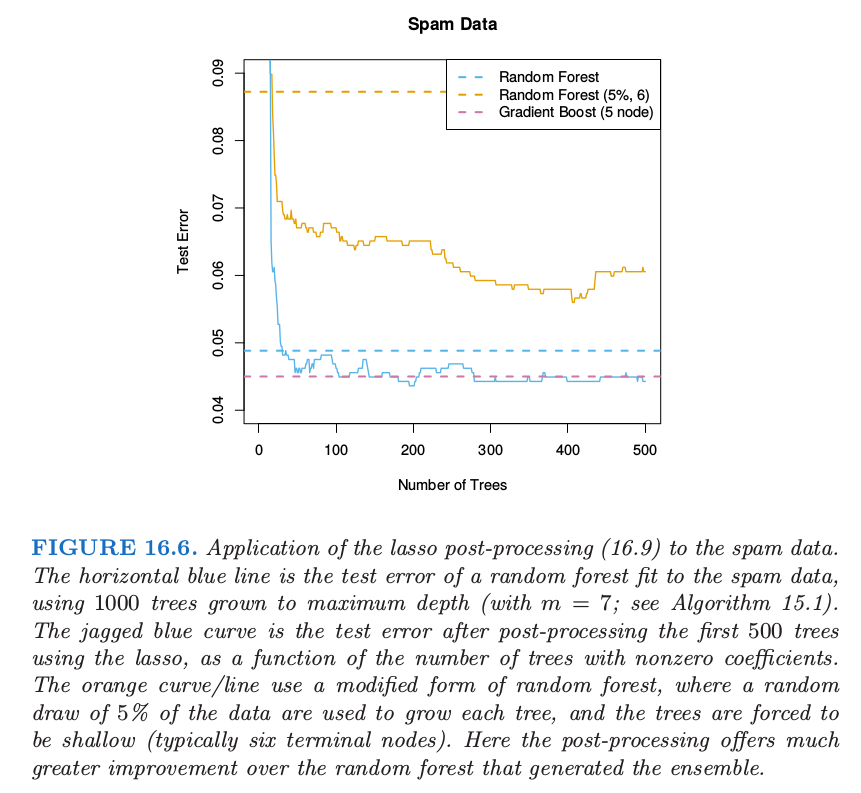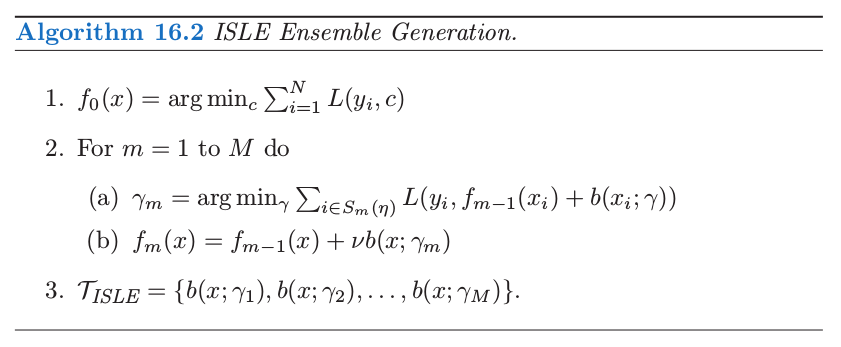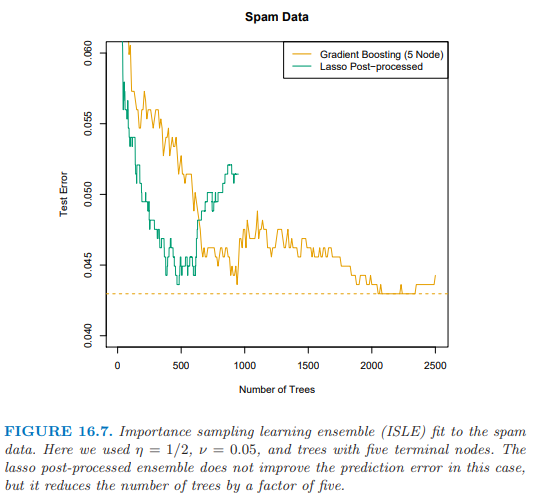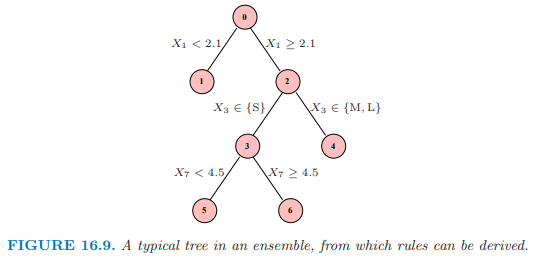# 16.3 学习集成¶

Friedman and Popescu (2003)1 提出混合的方式，将这个过程分解为下面两步：

• 从训练集中导出有限的基函数字典集 $\cT_L=\{T_1(x), T_2(x),\ldots, T_M(x)\}$;
• 通过在字典集中拟合 lasso 路径来构造 $f_\lambda(x)$ 函数族．## 学习一个好的集成¶

Friedman and Popescu (2003)1数值积分 (numerical quadrature)重要性采样 (importance sampling) 中获得启发．他们将未知的函数看成如下的积分

• $\sigma$ 太窄意味着许多 $b(x;\gamma_m)$ 很接近或者近似为 $b(x;\gamma^*)$；
• $\sigma$ 太宽意味着 $b(x;\gamma_m)$ 过于分散，但是可能包含其他许多无关的情形．

Friedman and Popescu (2003) 1 提出采用 sub-sampling 作为引入随机性的机制，得到了他们的集成生成算法 16.2．$S_m(\eta)$ 指的是大小为 $N\cdot \eta (\eta\in [0, 1])$ 的训练观测值的子样本，一般是无放回采样． 他们的模拟建议取 $\eta\le 1/2$，并且对于大 $N$ 取 $\eta\sim 1/\sqrt{N}$．降低 $\eta$ 会提高随机性，也因此提高宽度 $\sigma$．参数 $\nu\in[0, 1]$ 对随机过程引入 记忆 (memory)；$\nu$ 越大，越能避免 $b(x;\gamma)$ 与之前找到的一致．很多熟悉的随机化模式都是算法 16.2 的特殊情形：

• Bagging：$\eta=1$，但是是有放回地采样，并且有 $\nu=0$．Friedman and Hall (2007)2 认为 $\eta=\frac 12$ 时无放回抽样等价于 $\eta=1$ 时的采样，并且前者更有效．
• Random forest 的采样也类似，只是通过分离变量的选择引入了更多的随机性．在算法 16.2 中降低 $\eta<\frac 12$ 与随机森林中减低 $m$ 有类似的效果，但是不会有 15.4.2 节 中讨论的潜在的偏差问题．
• Gradient boosting，收缩为 \eqref{10.41} 时采用 $\eta=1$，但是一般不会得到充分大的 $\sigma$．
• Stochastic gradient boosting (Friedman, 19993) 完全遵循这个方法．

Recall## 规则集成 (Rule Ensembles)¶• 模型空间被增大了，并且可以得到更好的表现．
• 规则比树更好解释，所以有更简单模型的潜力．
• 经常通过分开加入变量 $X_j$ 来增广 ${\cal T}_{RULE}$，因此允许 ensemble 能够很好地建立线性模型．

Friedman and Popescu (2008)4 在一系列例子中展示了这个过程的能力，包括模拟例子 \eqref{16.13}．图 16.10 展示了从该模型得到的 20 次实现的真实模型的均方误差的箱线图．采用 Rulefit 软件所有模型都能拟合，这个软件可以在 ESL 主页找到，它采用自动的模式运行．

1. Friedman, J. and Popescu, B. (2003). Importance sampled learning ensembles, Technical report, Stanford University, Department of Statistics. 链接

2. Friedman, J. and Hall, P. (2007). On bagging and nonlinear estimation, Journal of Statistical Planning and Inference 137: 669–683.

3. Friedman, J. (1999). Stochastic gradient boosting, Technical report, Stanford University.

4. Friedman, J. and Popescu, B. (2008). Predictive learning via rule ensembles, Annals of Applied Statistics, to appear.